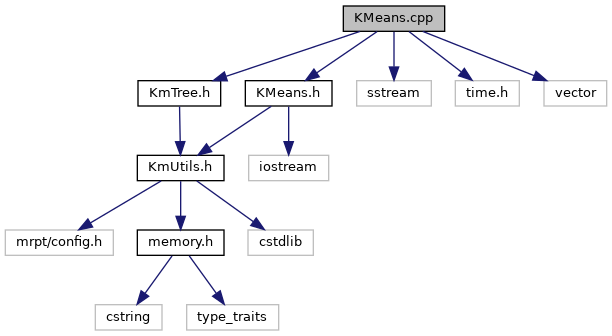MRPT  1.9.9
kmeans++/KMeans.cpp File Reference
`#include "KMeans.h"`
`#include "KmTree.h"`
`#include <sstream>`
`#include <time.h>`
`#include <vector>`
Include dependency graph for kmeans++/KMeans.cpp:Go to the source code of this file.

## Macros

#define LOG(verbose, text)

## Functions

void AddKMeansLogging (std::ostream *out, bool verbose)

void ClearKMeansLogging ()

static double GetSeconds ()

static void RunKMeansOnce (const KmTree &tree, int n, int k, int d, Scalar *points, Scalar *centers, Scalar *min_cost, Scalar *max_cost, Scalar *total_cost, double start_time, double *min_time, double *max_time, double *total_time, Scalar *best_centers, int *best_assignment)

void LogMetaStats (Scalar min_cost, Scalar max_cost, Scalar total_cost, double min_time, double max_time, double total_time, int num_attempts)

Scalar RunKMeans (int n, int k, int d, Scalar *points, int attempts, Scalar *ret_centers, int *ret_assignment)

Scalar RunKMeansPlusPlus (int n, int k, int d, Scalar *points, int attempts, Scalar *ret_centers, int *ret_assignment)

## Variables

static vector< ostream * > gLogOutputs

static vector< ostream * > gVerboseLogOutputs

## ◆ LOG

 #define LOG ( verbose, text )
Value:
{ \
vector<ostream*>& outputs = \
if (outputs.size() > 0) \
{ \
ostringstream string_stream; \
string_stream << text; \
for (int i = 0; i < (int)outputs.size(); i++) \
*(outputs[i]) << string_stream.str(); \
} \
}
static vector< ostream * > gVerboseLogOutputs
static vector< ostream * > gLogOutputs

Definition at line 24 of file kmeans++/KMeans.cpp.

Referenced by LogMetaStats(), RunKMeans(), RunKMeansOnce(), and RunKMeansPlusPlus().

## Function Documentation

 void AddKMeansLogging ( std::ostream * out, bool verbose )

Definition at line 36 of file kmeans++/KMeans.cpp.

References gLogOutputs, and gVerboseLogOutputs.

## ◆ ClearKMeansLogging()

 void ClearKMeansLogging ( )

Definition at line 41 of file kmeans++/KMeans.cpp.

References gLogOutputs, and gVerboseLogOutputs.

## ◆ GetSeconds()

 static double GetSeconds ( )
static

Definition at line 48 of file kmeans++/KMeans.cpp.

Referenced by RunKMeans(), RunKMeansOnce(), and RunKMeansPlusPlus().

## ◆ LogMetaStats()

 void LogMetaStats ( Scalar min_cost, Scalar max_cost, Scalar total_cost, double min_time, double max_time, double total_time, int num_attempts )

Definition at line 103 of file kmeans++/KMeans.cpp.

References LOG.

Referenced by RunKMeans(), and RunKMeansPlusPlus().

## ◆ RunKMeans()

 Scalar RunKMeans ( int n, int k, int d, Scalar * points, int attempts, Scalar * ret_centers, int * ret_assignment )

Definition at line 118 of file kmeans++/KMeans.cpp.

## ◆ RunKMeansOnce()

 static void RunKMeansOnce ( const KmTree & tree, int n, int k, int d, Scalar * points, Scalar * centers, Scalar * min_cost, Scalar * max_cost, Scalar * total_cost, double start_time, double * min_time, double * max_time, double * total_time, Scalar * best_centers, int * best_assignment )
static

## ◆ RunKMeansPlusPlus()

 Scalar RunKMeansPlusPlus ( int n, int k, int d, Scalar * points, int attempts, Scalar * ret_centers, int * ret_assignment )

Definition at line 177 of file kmeans++/KMeans.cpp.

## ◆ gLogOutputs

 vector gLogOutputs
static

Definition at line 22 of file kmeans++/KMeans.cpp.

Referenced by AddKMeansLogging(), and ClearKMeansLogging().

## ◆ gVerboseLogOutputs

 vector gVerboseLogOutputs
static

Definition at line 23 of file kmeans++/KMeans.cpp.

Referenced by AddKMeansLogging(), and ClearKMeansLogging().

 Page generated by Doxygen 1.8.14 for MRPT 1.9.9 Git: 7d5e6d718 Fri Aug 24 01:51:28 2018 +0200 at lun nov 2 08:35:50 CET 2020